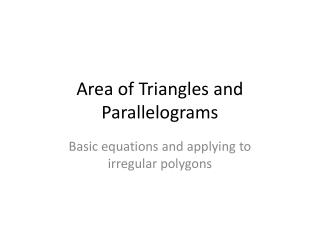DownloadDownload PresentationArea of Triangles and Parallelograms

# Area of Triangles and Parallelograms

Télécharger la présentation## Area of Triangles and Parallelograms

- - - - - - - - - - - - - - - - - - - - - - - - - - - E N D - - - - - - - - - - - - - - - - - - - - - - - - - - -
##### Presentation Transcript

1. Area of Triangles and Parallelograms Basic equations and applying to irregular polygons

2. Rectangles and Parallelograms These two go together because the are very similar shapes Base time height Remember the height is the perpendicular segment between two bases Rectangle – sides are perpendicular Parallelogram – need to have height or calculate the height, may be outside the parallelogram

3. Triangles Half the area of the Rectangle or parallelogram it would fit into Height or Altitude – perpendicular segment from vertex to side opposite, could be outside the triangle

4. Trapezoid Think of this as two triangles – they have different bases Sum of two bases times height divided by 2 Can’t always divide trapezoid into a rectangle and two triangles, the end triangles may not be the same

5. Kite Split into 2 congruent triangles using the diagonals Area of one triangle times 2